# Alumina 3 Bayer Species Model

Jump to navigation Jump to search

Navigation: Main Page -> Models -> Alumina Models

## General Description

The Alumina3 Bayer species model is used to calculate the properties of fluids within an Alumina project using equations defined in the public domain.

The unit operation Precipitation3 requires Alumina3 Bayer species model to function properly. Please see Precipitation3 for documentation.

Note: The Precipitation Projects and Digestion Projects, which are distributed with SysCAD in the Examples Folder, demonstrate the use of the Alumina 3 Bayer species model in SysCAD projects.

## Required Chemical Compounds

The following species are referenced by the Alumina3 set of models and are required in the species database and configuration file, except for Sodium Fluoride, which is optional. If it is present in the database, its effects will be included in the correlation calculations.

 VAPOUR H2O(g) - Water Vapour LIQUIDS H2O(l) - Water NaAl[OH]4(aq) - Sodium Aluminate NaOH(aq) - Caustic Soda Na2CO3(aq) - Sodium Carbonate Na2SO4(aq) - Sodium Sulphate NaCl(aq) - Sodium Chloride Na2SiO3(aq) - Sodium Silicate NaF(aq) - Sodium Fluoride (Optional) ORGANICS Na2C2O4(aq) - Sodium Oxalate Na2C5O7(aq) - Organic compound representation SOLIDS Al[OH]3(s) - Gibbsite (THA) Al2O3(s) - Alumina Solid Na2O(s) - Occluded Soda NaOH*(s) - Bound Soda (Optional) NOTE: This is only used when using the Precipitation3 unit operation. Na2C5O7*(s) - Bound Organics (Optional) NOTE: This is only used when using the Precipitation3 unit operation.

Note: Sodium Fluoride is not required but will be included in total sodium calculation if present.

## Model Theory

Please see Species Model Theory for the equations used.

All of the properties that are not explicitly calculated by this model are calculated using the Standard Species Model.

Please see General Bayer Data for some general discussion topics.

## Bayer Property Calculation Method

Some Bayer properties have different calculation methods, user may select which method to use in the project. Please note that the method selected is Global to the project.

The method and Constants selection can be selected from Plant Model - Global Tab: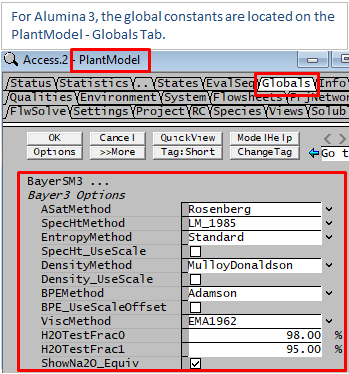Please see Global Properties Calculation Method Selection for more information.

## Selecting a Bayer Species Model in a Feeder

The user must have a project that includes a Bayer species model in the configuration file as shown below.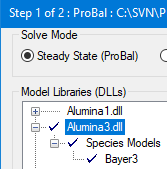It is then possible to select the Bayer model (either Bayer3 or a Client Specific Bayer model).

• On the Feeder access window there is a field 'SpModel.Reqd' where the user may select the model, as shown below: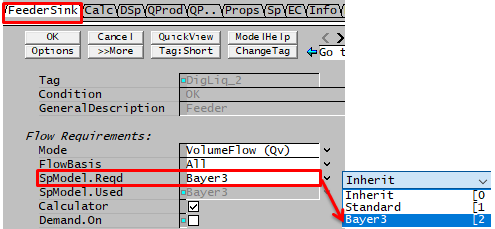Tag / Symbol Input / Calc Description SpModel.Reqd Inherit Model used follows upstream units. Standard All the variables are calculated using the Mass Weighted Mean of the species. A Bayer Model ('A Bayer Model' will be the Bayer model included in the configuration file. In the view above this is 'Bayer3'). Density, Cp, Boiling Point Elevation, etc calculated using the equations defined for that particular Bayer species model.

## Bayer Liquor Calculator

When defining a Bayer Liquor stream, it is possible to have SysCAD calculate the feed composition based on user defined Bayer species concentrations and ratios.

This is done in the Feeder unit - but only in a 'True' feeder, i.e. not connected to a sink on a different flowhseet:

This is shown in the images below: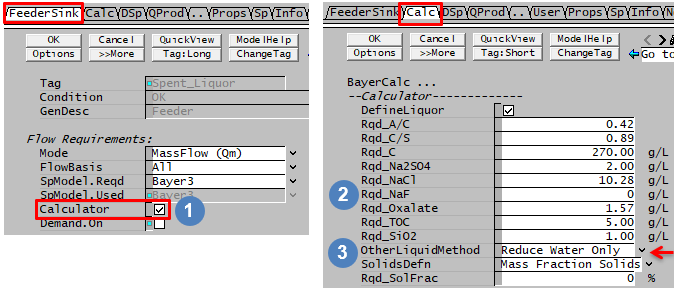For more information on the feed calculator variables, please see Calculator - Bayer Feed Calculator.

The calculated feed composition will be set into the DSp tab. The variables set by the calculator are indicated by the yellow background.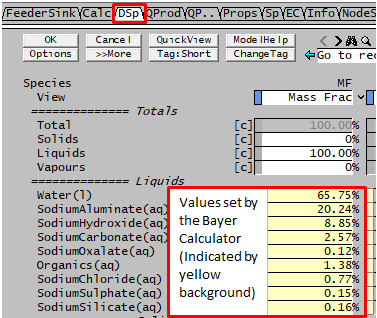NOTE:

1. If the calculator is enabled, the user may NOT manually change the feed composition on the DSp tab. All changes must be made on the Calc tab.
2. The calculator will calculate the required concentration based on the required alumina 3 liquid species, if other liquid species are present and contain user specified values, the final concentrations might not match the calculator specifications. Please select one of the "OtherLiquidMethod" to help reduce this error.
3. The user may enable the calculator once to get an idea of the values, and then turn the calculator off to manually change the DSp tab values, if required.
4. If the feeder is connected to a sink on another flowsheet, the calculated values are ignored.
5. The Feed Calculator maybe different for different species model, please see Alumina 1 vs Alumina 3 for the difference between these two generic species models.

## Size Data

If the Bayer stream has Alumina Particle Size information for use in Precipitation3 unit model, see the relevant quality model documentation for Specific Surface Area (SSA) or Size Distribution (PSD) for display and configuration information.

## Data Sections

### Global Properties Calculation Method Selection

 Tag / Symbol Input / Calc Description Menu Command View - Plant Model - Globals Tab - Bayer Properties: ASatMethod Rosenberg Saturated Alumina concentration. See Saturated Alumina Concentration for equations used. RosenbergUser This uses the same equation as above, but user are able to adjust all the constants in the equation manually. SpecHtMethod LM_1985 See Heat Capacity Calculations for equations used. Mulloy Donaldson See Heat Capacity Calculations for equations used. EntropyMethod Standard Entropy is calculated by the Standard Species Model using mass weighted mean. LM_1985 Based on the LM1985 specific heat. Only available in Build 137. Please see Entropy Calculations for equation used. SpecHt_UseScale Tickbox This allows user to add in a scaling factor for the calculated Bayer Specific Heat value, useful when tuning project to match plant conditions. SpecHt_Factor Input This field is only visible if the SpecHt_UseScale tick box is selected. Used to adjust the calculated Bayer Specific Heat value linearly. DensityMethod MulloyDonaldson See Density Calculations for equations used. MulloyDonaldsonUser This uses the same equation as above, but user are able to adjust all the constants in the equation manually. Density_UseScale Tickbox This allows user to add in a scaling factor for the Bayer Liquor Density value, useful when tuning project to match plant conditions. Density_Factor Input This field is only visible if the Density_UseScale tick box is selected. Used to adjust the calculated Bayer Density value linearly. BPEMethod Dewey This is the original method implemented in SysCAD. This method becomes inaccurate in high caustic concentration range. Adamson This method is more accurate in the high caustic concentration range. See Boiling Point Elevation for equations used. BPE_UseScaleOffset Tickbox This enables a global correction (Scale Factor and Offset) to the calculated BPE value. The resulting BPE has a lower limit 0 degrees. BPE_Factor Input This field is only visible if the BPE_UseScaleOffset option is selected. Scale for global correction value for Boiling Point Elevation where final BPE = Calculated BPE * BPE Factor + BPE Offset. Result is limited to a minimum of zero. BPE_Offset Input This field is only visible if the BPE_UseScaleOffset option is selected. Offset for global correction value for Boiling Point Elevation where final BPE = Calculated BPE * BPE Factor + BPE Offset. Result is limited to a minimum of zero. ViscMethod EMA1962 Liquid Viscosity. Limitation apply, see Viscosity for equations used. Alexandrov Caustic solution viscosity. See Viscosity for equations used. This method is only available in SysCAD 93. H2OTestFrac0 Input This is used to handle very dilute concentration in the stream. If the Water Fraction in the stream is higher than this value, then the stream property will be calculated using the Standard Species Model. The maximum value for this is 99.99%. H2OTestFrac1 Input This is used to handle very dilute concentration in the stream. If the Water Fraction in the stream is lower than this value, then the stream property will be calculated using the Bayer3 Species model. For water fraction higher than this value but lower than H2OTestFrac0, a proportional calculation will be performed using both the Standard Species Model and the Bayer3 Species model. ShowNa2O_Equiv Input Selecting this option will add to the display list of Bayer liquor properties variables expressed on Na2O basis.

### Calculator - Bayer Feed Calculator

 Tag (Long/Short) Input / Calc Description Maximum Bayer Liquor Design Values DefineLiquor Check Box If this box is checked, the Feeder will automatically calculate the make-up of the feed stream, based on the variables supplied below. Rqd_A/C / A/C_Reqd Input The required ratio of A:C in the Feed stream, where A is Sodium Aluminate NaAl[OH]4 concentration, expressed as grams of Al2O3 /L liquor and C is Caustic Soda concentration in NaOH + NaAl[OH]4, expressed as grams Na2CO3/L liquor. 0.962003767 (MWAl2O3/MWNa2CO3) Rqd_C/S / C/S_Reqd Input The required ratio of C:S in the Feed stream, where C is Caustic Soda concentration in NaOH + NaAl[OH]4, expressed as grams Na2CO3/L liquor and S is C+ Na2CO3 concentration, expressed as grams Na2CO3/L liquor. A minimum ratio of 0.1 is applied, however depending on other required concentrations a low A/C ratio can result in an unachievable mixture. 1.0 Rqd_C / C_Reqd Input The required Caustic Soda concentration in NaOH + NaAl[OH]4, expressed as grams Na2CO3/L liquor @ 25°C. 550.0 Rqd_Na2SO4 / Na2SO4_Reqd Input Required concentration of Na2SO4 in g/L @ 25°C 550.0 Rqd_NaCl / NaCl_Reqd Input Required concentration of NaCl in g/L @ 25°C 550.0 Rqd_NaF / NaF_Reqd Input Required concentration of NaF in g/L @ 25°C. Only appears if NaF is present as a species. 50.0 Rqd_Oxalate / Oxalate_Reqd Input Required concentration of oxalate in g/L @ 25°C. 550.0 Rqd_TOC / TOC_Reqd Input Required total organics [Na2C5O7(aq) + Na2C2O4(aq)] concentration in the stream expressed as grams of elemental carbon /L liquor @ 25°C. This determines the non oxalate organics. If the specified oxalate organics result in a higher TOC than specified, non oxalate organics are set to zero. 200.0 Rqd_SiO2 / SiO2_Reqd Input Required concentration of Na2SiO3 expressed as grams SiO2 /L liquor @ 25°C 550.0 The ZeroOtherLiqs tickbox or OtherLiquidMethod list box is only visible when other liquid species in addition to the Required alumina 3 liquid species are present in the project. The ZeroOtherLiqs tickbox is only available in Build135.13583 through to Build137.22042. This tick box has been replaced by OtherLiquidMethod drop-list in Build 137.22043. ZeroOtherLiqs Tickbox The number of compounds present will be shown in () to the right of the tick box. Selecting this tick box will set all the liquid flows not used in the calculation to zero, this will ensure the output from Bayer DefineLiquor will match the specified concentrations. If this box is NOT ticked, User can manually specify composition for the other liquids, the amount of liquids specified will be added to the Total from the Bayer DefineLiquor Calculation. That means, the end result may not match the specified concentrations. N/A OtherLiquidMethod Zero Other Liqs Selecting this method will set all the liquid flows not used by the bayer calculation to zero, this will ensure the output from Bayer DefineLiquor will match the specified concentrations. N/A Original (This method is NOT recommended, it is available only for backward compatibility.) User can manually specify composition for the other liquids, the amount of liquids specified will be added to the Total from the Bayer DefineLiquor Calculation. That means, the end result may not match the specified concentrations. N/A Reduce Bayer Liqs User can manually specify composition for the other liquids. The calculated bayer liquor composition will be adjusted to offset the user specified values. The end result may not match the specified concentrations. N/A Reduce Water Only (This is the recommended method). User can manually specify composition for the other liquids. The calculated water composition will be adjusted to offset the user specified values. The end result will match the specified concentrations. N/A SolidsDefn Mass Fraction Solids The required solids are specified by solids mass fraction. N/A Total Solids Conc The required solids are specified by total solids concentration. N/A SolFracReqd / Rqd_SolFrac Input The mass fraction of solids in the feed. The proportion of the different solid species is maintained. If there are no solid species specified then THA is assumed. 0.99 SolConcReqd / Rqd_SolConc Input The concentration (at 25°C) of solids in the liquor. The proportion of the different solid species is maintained. If there are no solid species specified then THA is assumed. 1600.0

### Bayer Properties Data

A number of these will be deprecated in 9.2 since they are unused or their use has proved confusing. In particular there are a number of organic quantities (TOS, TOOC etc) that fall into this category.

 Tag | Symbol Input or Calc Description Bayer Liquor Values @ 25°C A | AluminaConc Calc NaAl[OH]4 concentration expressed as Al2O3 @ 25°C. (g/L liquor) C | CausticConc Calc NaOH + NaAl[OH]4 concentration, expressed as grams Na2CO3/L liquor @ 25°C. S | SodaConc Calc NaOH + NaAl[OH]4 + Na2CO3 concentration, expressed as grams Na2CO3/L liquor @ 25°C. A/C Calc Ratio of A to C C/S Calc Ratio of C to S Cl/C Calc Ratio of Cl to C TOC@25 Calc Total Organic Carbon concentration, as carbon equivalent. Grams of [Na2C5O7*5 + Na2C2O4*2] / L liquor @ 25°C. TOS@25 (Deprecated) Calc Total Organic Soda concentration, grams of [Na2C5O7 + Na2C2O4] / L liquor @ 25°C. SodiumCarbConc Calc Sodium Carbonate concentration SolidConc25 Calc Expressed as grams of solids / L Slurry @ 25°C. FC | FreeCaustic Calc Free Caustic, Caustic Concentration excluding the amount asscoiated with NaAl[OH]4, expressed as g/L of Na2CO3. Sulphate*@25 Calc Sodium Sulphate concentration @ 25°C Chloride*@25 Calc Sodium Chloride concentration @ 25°C Oxalate*@25 Calc Oxalate Concentration @ 25°C Silica*@25 Calc Silicate Concentration as SiO2 @ 25°C Silica/C Calc Silica to Caustic ratio times 1000. NaOnCS Calc Ratio of Total Sodium (TotalNa) to Caustic Concentration (C) TOS/TOC (Deprecated) Calc Ratio of TOS to TOC (Total Organic soda expressed as Na2CO3 / Total Organic Carbon expressed as Carbon) Concentration @ 25°C, as Na2CO3 equivalent Organate@25 Calc Grams of organic Na2C5O7(l) / L liquor @ 25°C. Oxalate@25 Calc Grams of oxalate Na2C2O4(l) / L liquor @ 25°C. NaCl@25 Calc Grams of NaCl(l) / L liquor @ 25°C. Na2SO4@25 Calc Grams of Na2SO4(l) / L liquor @ 25°C. TotalNa@25 Calc Total sodium as per TNa Equation in Model Theory section, but expressed as g/L liquor @ 25°C. Other properties @ 25°C LRho25 | LiquidDensity@25 Calc The liquor density @ 25°C. SLRho25 | SlurryDensity@25 Calc The slurry density @ 25°C. LQv25 | LVolFlow25 Calc Liquor Volumetric Flowrate @25°C. SLQv25 | SLVolFlow25 Calc Slurry Volumetric Flowrate @25°C. Bayer Liquor Values @ 25 °C, as Na2O Equivalent NOTE: This group of variables is only visible if the View|PlantModel|Globals - ShowNa2O_Equiv tickbox is selected. C_Na2O Calc NaOH + NaAl[OH]4 concentration, expressed as grams Na2O/L liquor @ 25°C. S_Na2O Calc NaOH + NaAl[OH]4 + Na2CO3 concentration, expressed as grams Na2O/L liquor @ 25°C. TotalNa@25_Na2O Calc Total Sodium, expressed as grams Na2O/L Liquor @ 25°C. RP | A/C_Na2O Calc Ratio of A : C expressed as Na2O. RPSat | A/C_Sat_Na2O Calc Ratio of ASat : C@25 expressed as Na2O. Bayer Liquor Values @ Temperature A@T | AluminaConcT Calc Al2O3 concentration @ Temperature (g/L liquor) C@T | CausticConcT Calc NaOH + NaAl[OH]4 concentration, expressed as grams Na2CO3/L liquor @ Temperature. S@T | SodaConcT Calc NaOH + NaAl[OH]4 + Na2CO3 concentration, expressed as grams Na2CO3/L Liquor @ Temperature. TOC@T Calc Total organic carbon concentration as Carbon equivalent. Grams of [Na2C5O7*5 + Na2C2O4*2] / L liquor @ Temperature. SodiumCarbConc@T Calc Sodium Carbonate concentration @ Temperature. SolidConc@T Calc Expressed as grams of solids / L Slurry @ Temperature. BPE@T | BoilPtElev Calc Boiling Point Elevation calculated as a function of stream temperature. Note: this differs to the BPE value displayed under the Saturation section as BPE is calculated as a function of pressure. The two values and BPE@P will be the same if the stream is at saturated conditions. BPE@P | BoilPtElev@P Calc Boiling Point Elevation calculated as a function of stream pressure. Note: this is the same as the BPE value displayed under the Saturation section. BPE@T will be the same as BPE@P if the stream is at saturated conditions. Bayer Liquor Values @ T, as Na2O EquivalentNOTE: This group of variables is only visible if the View|PlantModel|Globals - ShowNa2O_Equiv tickbox is selected. CausticConc@T_Na2O Calc NaOH concentration, expressed as grams Na2O/L liquor @ Temperature. SodaConc@T_Na2O Calc NaOH plus Na2CO3 concentration, expressed as grams Na2O/L liquor @ Temperature. TotalNa@T_Na2O Calc Total Sodium, expressed as grams Na2O/L Liquor @ Temperature. Bayer Liquor Solubility Properties @ T ASat | A_Saturation Calc The saturated Alumina concentration @ stream temperature. (Note: As described in Model Theory, concentrations used in the correlation are referenced to 25°C). AConcEquil | AConcEquilibrium Calc Alumina Concentration (A) at Equilibrium, ie accounting for changes in composition to liquor if equilibrium saturation is reached. CConcEquil | CConcEquilibrium Calc Caustic Concentration (C) at Equilibrium, ie accounting for changes in composition to liquor if equilibrium saturation is reached. A/CSat | A/C_Saturation Calc The ratio of ASat : C. (Note: Concentrations used in ASat correlation are referenced to 25°C, so ratio is ASat : C@25). SSN_Ratio Calc The ratio of A : ASat which is the same as ratio of fields 'A/C' : 'A/CSat'. (Note: Concentrations used in ASat correlation are referenced to 25°C, so ratio is A@25 : ASat). A_ASat_Diff Calc The difference between Alumina Concentration @ temperature and Alumina Saturation Concentration. So result is fields 'A@T' - 'ASat'. I | IonicStrength Calc The Ionic strength. See model theory under Alumina Saturation for equation. OxalateEq | OxalateEquilibrium Calc The Oxalate Equilibrium value. See Model Theory for equation. THA.Qm | THAMassFlow Calc The mass flowrate of THA - Al[OH]3(s). THA.Rho | THADens Calc The density of THA - Al[OH]3(s).